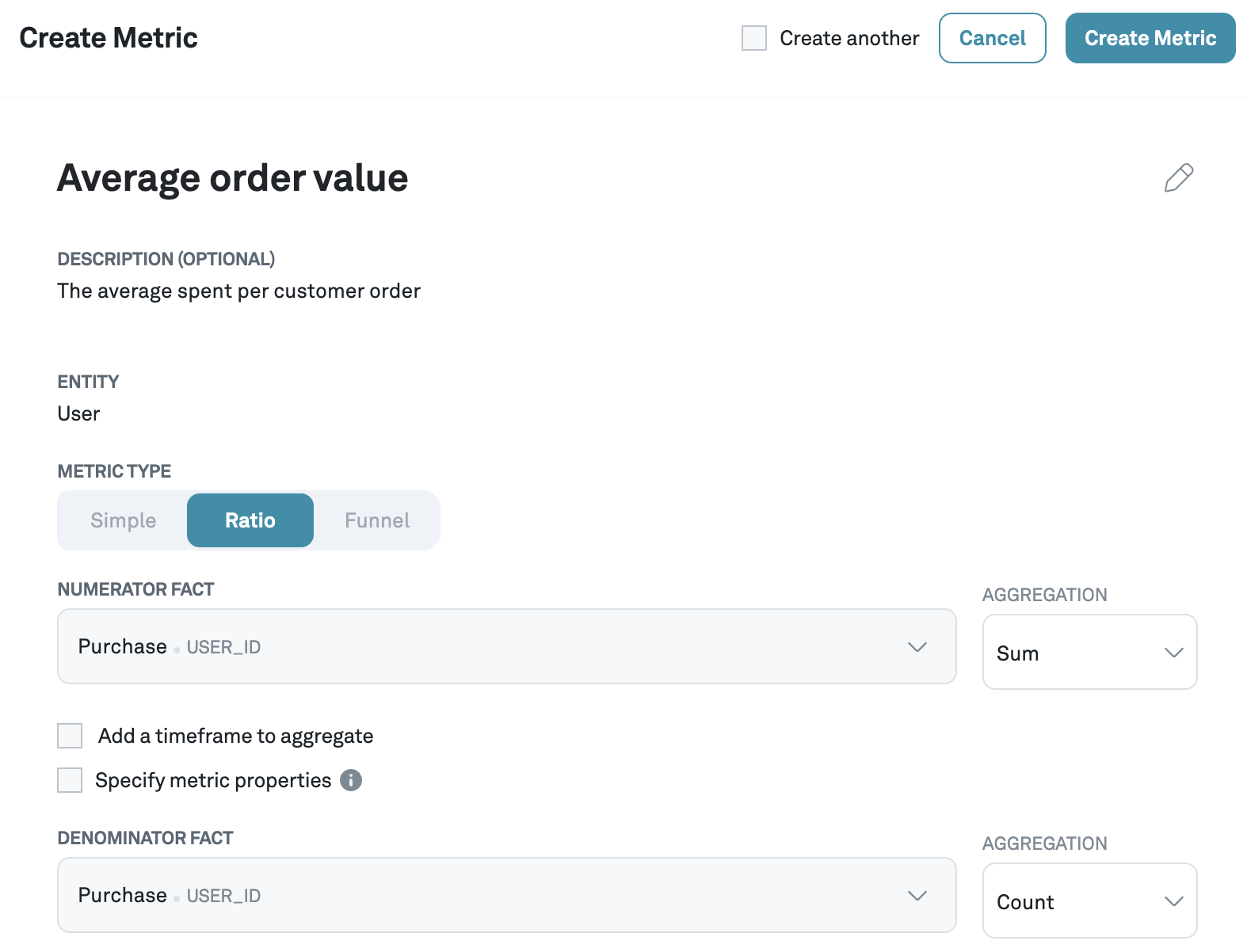# Ratio metrics

Ratio metrics allow you to calculate ratio of two simple metrics, providing deeper insights into the relative performance of variations. This enables a more nuanced analysis of experimental results, allowing businesses to understand the impact of changes in a more comprehensive manner.

For example, consider an average order value metric, which is created by dividing revenue (sum of prices) by number of orders (sum of items purchased or count of prices).

$\text{Average order value} = \frac{\text{Revenue}}{\text{Number of orders}}$
note

Ratio metrics are computed by first computing averaged values for the numerator and the denominator, and then dividing them to compute the ratio, rather than computing the ratio on a user level and then taking the average.

info

We use the Delta method to compute valid statistical results for ratio metrics.

## Creating a ratio metric​

Creating a ratio metric follows the same approach as creating a simple metric. Of course, the difference is that for a ratio metric, you have to select both a fact and aggregation for numerator and denominator.